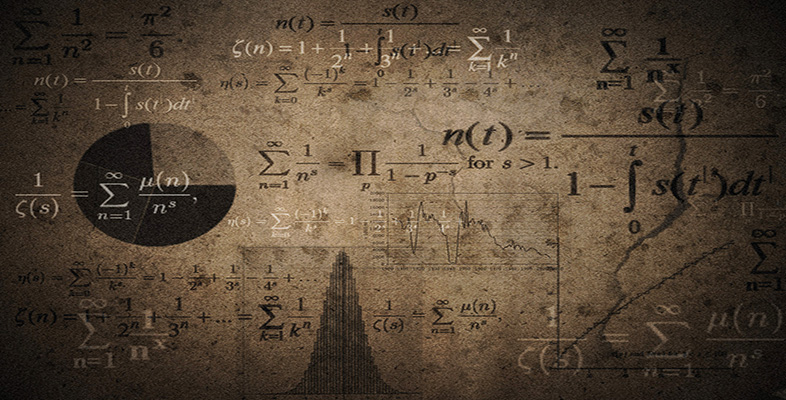Language, notation and formulas

This free course is available to start right now. Review the full course description and key learning outcomes and create an account and enrol if you want a free statement of participation.

Free course

# 3.1.1 Try some yourself

## Activity 12

Write the folowing as word formulas and then shorten them

• (a) The area of a square is its length squared.

• (b) The area of a rectangle is its length times its breadth.

• (c) The area of a triangle is half its base times its height

• (a) The area of a square = (length)2 = l2 where l stands for length.

• (b) The area of a rectangle = (length) × (breadth) = l × b or lb where l stands for length and b for breadth.

• (c) The area of a triangle is(base) × (height) =b × h orbh where b stands for base and h for height

(Note it is important to be clear about what each letter represents).

MU120_4M6